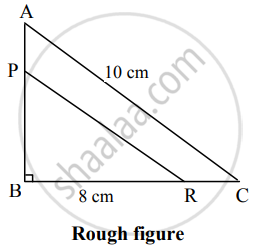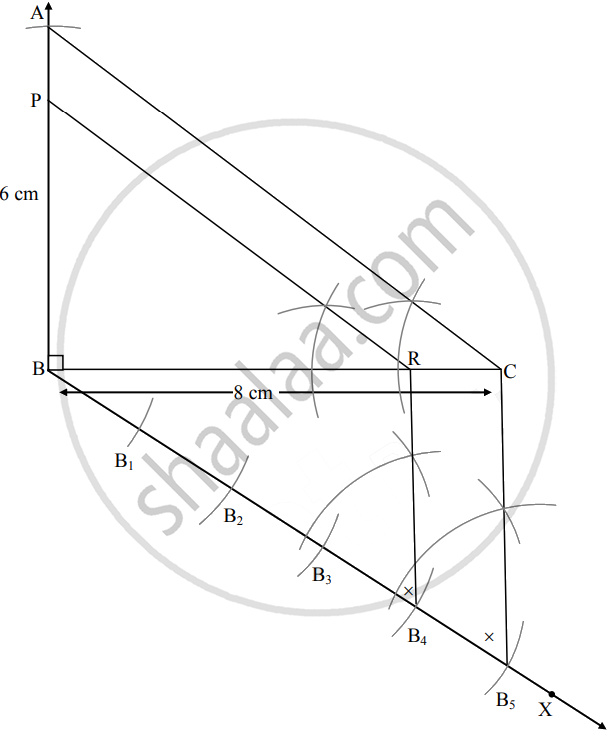# ΔABC ~ ΔPBR, BC = 8 cm, AC = 10 cm , ∠B = 90°, BCBR=54 then construct ∆ABC and ΔPBR - Geometry

Diagram

ΔABC ~ ΔPBR, BC = 8 cm, AC = 10 cm , ∠B = 90°, "BC"/"BR" = 5/4 then construct ∆ABC and ΔPBR

#### SolutionAnalysis: In ∆ABC, ∠B = 90°    ......[Given]

∴ AC2 = AB2 + BC2    ......[Pythagoras theorem]

∴ 102 = AB2 + 82

∴ AB2 = 100 – 64

∴ AB2 = 36

∴ AB = 6 cm     ......[Taking square root of both sides]Steps of construction:

1. Draw seg BC of length 8 cm.
2. Take ∠B as 90° and draw an arc of 6 cm on it. Name the point as A.
3. Join seg AC to obtain ∆ABC.
4. Draw ray BX such that ∠CBX is an acute angle.
5. Locate points B1, B2, B3, B4, B5 on ray BX such that, BB1 = B1B2 = B2B3 = B3B4 = B4B5.
6. Join point C and B5.
7. Through point B4 draw a line parallel to seg CB5 which intersects seg BC at point R.
8. Draw a line parallel to AC through R to intersect line AB at point P.
∆PBR is the required triangle similar to ∆ABC.
Concept: Division of a Line Segment
Is there an error in this question or solution?

Share